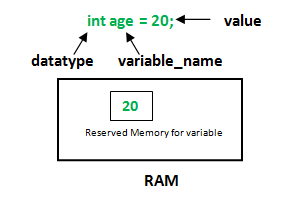GeeksforGeeks App
Open AppBrowser
Continue

# How to avoid Compile Error while defining Variables

Variables: A variable is the name given to a memory location. It is the basic unit of storage in a program.

• The value stored in a variable can be changed during program execution.
• A variable is only a name given to a memory location, all the operations done on the variable effects that memory location.
• All the variables must be declared before use.

How to declare variables?
We can declare variables in common languages (like C, C++, Java etc) as follows:```where:
datatype: Type of data that can be stored in this variable.
variable_name: Name given to the variable.
value: It is the initial value stored in the variable.
```

How to avoid errors while creating variables?

1. The identifier is undeclared: In any programming language, all variables have to be declared before they are used. If you try to use the name of a such that hasn’t been declared yet, an “undeclared identifier” compile-error will occur.

Example:

 `#include `` ` `int` `main()``{`` ` `    ``printf``(``"%d"``, x);``    ``return` `0;``}`

Compile Errors:

```prog.c: In function 'main':
prog.c:5:18: error: 'x' undeclared (first use in this function)
printf("%d", x);
^
prog.c:5:18: note: each undeclared identifier is reported
only once for each function it appears in
```
2. No initial value is given to the variable: This error commonly occurs when the variable is declared, but not initialized. It means that the variable is created but no value is given to it. Hence it will take the default value then. But in C language, this might lead to error as this variable can have a garbage value (or 0) as its default value. In other languages, 0 will be its default value.

Example:

 `#include `` ` `int` `main()``{`` ` `    ``int` `x;`` ` `    ``printf``(``"%d"``, x);``    ``return` `0;``}`

Output:

```0
```
3. Using variable out of its Scope: Scope of a variable is the part of the program where the variable is accessible. Like C/C++, in Java, all identifiers are lexically (or statically) scoped, i.e.scope of a variable can be determined at compile time and independent of the function call stack.

Example:

 `#include `` ` `int` `main()``{`` ` `    ``{``        ``int` `x = 5;``    ``}`` ` `    ``printf``(``"%d"``, x);``    ``return` `0;``}`

Compile Errors:

```prog.c: In function 'main':
prog.c:5:18: error: 'x' undeclared (first use in this function)
printf("%d", x);
^
prog.c:5:18: note: each undeclared identifier is reported
only once for each function it appears in
```

How to Correct the above code: Declare the variable x before using it in the outer scope. Or you can use the already defined variable x in its own scope

Example:

 `#include `` ` `int` `main()``{`` ` `    ``{``        ``int` `x = 5;``        ``printf``(``"%d"``, x);``    ``}`` ` `    ``return` `0;``}`

Output:

```5
```
4. Creating a variable with an incorrect type of value: This arises due to the fact that values are implicitly or explicitly converted into another type. Sometimes this can lead to Warnings or errors.

Example:

 `#include `` ` `int` `main()``{`` ` `    ``char``* x;`` ` `    ``int` `i = x;`` ` `    ``printf``(``"%d"``, x);``    ``return` `0;``}`

Warning:

```prog.c: In function 'main':
prog.c:7:13: warning: initialization makes integer
from pointer without a cast [-Wint-conversion]
int i = x;
^
```

My Personal Notes arrow_drop_up